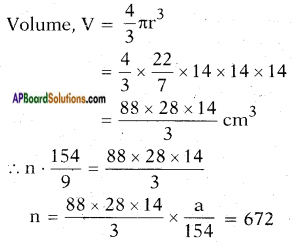# AP SSC 10th Class Maths Solutions Chapter 10 Mensuration Ex 10.4

AP State Board Syllabus AP SSC 10th Class Maths Textbook Solutions Chapter 10 Mensuration Ex 10.4 Textbook Questions and Answers.

## AP State Syllabus SSC 10th Class Maths Solutions 10th Lesson Mensuration Exercise 10.4

### 10th Class Maths 10th Lesson Mensuration Ex 10.4 Textbook Questions and Answers

Question 1.
A metallic sphere of radius 4.2 cm. is melted and recast into the shape of a cylinder of radius 6 cm. Find the height of the cylinder.
Given, sphere converted into cylinder.
Hence volume of the sphere = volume of the cylinder.
Sphere:
Volume V = $$\frac{4}{3}$$πr3
= $$\frac{4}{3}$$ × $$\frac{22}{7}$$ × 4.2 × 4.2 × 4.2
= 4 × 22 × 0.2 × 4.2 × 4.2
= 4 x 22 x 0.2 x 4.2 x 4.2
= 310.464
Cylinder:
Height h = h say
Volume = πr2h
= $$\frac{22}{7}$$ × 6 × 6 × h
= $$\frac{22 \times 36}{7} h$$
= $$\frac{792}{7} h$$
Hence, $$\frac{792}{7} h$$ = 310.464
h = $$\frac{310.464 \times 7}{792}$$ = 2.744cm
!! π can be cancelled on both sides i.e., sphere = cylinder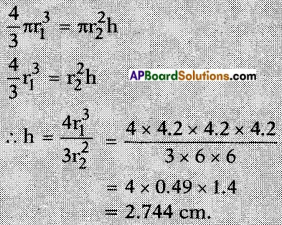Question 2.
Three metallic spheres of radii 6 cm., 8 cm. and 10 cm. respectively are melted together to form a single solid sphere. Find the radius of the resulting sphere.
Given : Radii of the three spheres r1 = 6 cm r2 = 8 cm r3 = 10 cm
These three are melted to form a single sphere.
Let the radius of the resulting sphere be ‘r’.
Then volume of the resultant sphere = sum of the volumes of the three small spheres.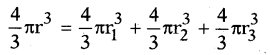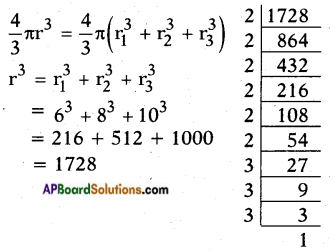∴ 1728 = (2 × 2 × 3) × (2 × 2 × 3) × (2 × 2 × 3)
r3 = 12 × 12 × 12
r3 = 123
∴ r = 12
Thus the radius of the resultant sphere = 12 cm

Question 3.
A 20 m deep well with diameter 7 m. is dug and the earth got by digging is evenly spread out to form a rectangu¬lar platform of base 22 m. × 14 m. Find the height of the platform.
Volume of earth taken out = πr2h
= $$\frac{22}{7}$$ × $$\frac{7}{2}$$ × $$\frac{7}{2}$$ × 20
= 770 m
Let height of plot form = H m.
∴ 22 × 14 × H = $$\frac{22}{7}$$ × $$\frac{7}{2}$$ × $$\frac{7}{2}$$ × 20
H = $$\frac{35}{14}$$ = $$\frac{5}{2}$$ = $$2 \frac{1}{2} \mathrm{~m}$$
∴ The height of the plat form is $$2 \frac{1}{2} \mathrm{~m}$$Question 4.
A well of diameter 14 m. is dug 15 m. deep. The earth taken out of it has been spread evenly all around it in the shape of a circular ring of width 7 m to form an embankment. Find the height of the embankment
Volume of the well = Volume of the embank
Volume of the cylinder = Volume of the embank
Cylinder :
Radius r = $$\frac{d}{2}$$ = $$\frac{14}{2}$$ = 7 cm
Height/depth, h = 15 m
Volume V = πr2h
= $$\frac{22}{7}$$ × 7 × 7 × 15
= 22 × 7 × 15
= 2310 m3
Embank: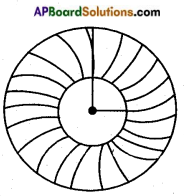Let the height of the embank = h m
= 7m + 7m = 14 m
Area of the base of the embank = (Area of outer circle) – (Area of inner circle)
= πR2 – πr2
= π(R2 – r2)
= $$\frac{22}{7}$$$$\left(14^{2}-7^{2}\right)$$
= $$\frac{22}{7}$$ × (14+7) × (14-7)
= $$\frac{22}{7}$$ × 21 × 7
= 462 m2
∴ Volume of the embank = Base area × height
= 462 × h = 462 h m3
∴ 462 h m3 = 2310 m3
h = $$\frac{2310}{462}$$ = 5 m.Question 5.
A container shaped like a right circular cylinder having diameter 12 cm. and height 15 cm. is full of ice-cream. The ice-cream is to be filled into cones of height 12 cm. and diameter 6 cm., having a hemispherical shape on the top. Find the number of such cones which can be filled with ice-cream.
Let the number of cones that can be filled with the ice-cream be ‘n’.
Then total volume of all the cones with a hemi spherical top = Volume of the ice-cream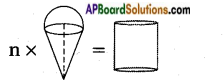Ice-cream cone = Cone + Hemisphere = πr2h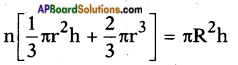Cone:
Radius = $$\frac{d}{2}$$ = $$\frac{6}{2}$$ = 3 cm
Height, h = 12 cm
Volume V = $$\frac{1}{3}$$πr2h
= $$\frac{1}{3}$$ × $$\frac{22}{7}$$ × 3 × 3 × 12
= $$\frac{22}{7}$$ × 36
= $$\frac{792}{7}$$
Hemisphere:
Radius = $$\frac{d}{2}$$ = $$\frac{6}{2}$$ = 3 cm
Volume V = $$\frac{2}{3}$$πr3
= $$\frac{2}{3}$$ × $$\frac{22}{7}$$ × 3 × 3 × 3
= $$\frac{44 \times 9}{7}$$
= $$\frac{396}{7}$$
∴ Volume of each cone with ice-cream = $$\frac{792}{7}$$ + $$\frac{396}{7}$$ = $$\frac{1188}{7}$$ cm3
Cylinder:
Radius = $$\frac{d}{2}$$ = $$\frac{12}{2}$$ = 6 cm
Height, h = 15 cm
Volume V = πr2h
= $$\frac{22}{7}$$ × 6 × 6 × 15
= $$\frac{22 \times 36 \times 15}{7}$$
= $$\frac{11880}{7}$$
∴ $$\frac{11880}{7}$$ = n × $$\frac{11880}{7}$$
⇒ n = $$\frac{11880}{7}$$ × $$\frac{7}{1188}$$ = 10
∴ n = 10.Question 6.
How many silver coins, 1.75 cm in diameter and thickness 2 mm., need to be melted to form a cuboid of dimensions 5.5 cm × 10 cm × 3.5 cm?
Let the number of silver coins needed to melt = n
Then total volume of n coins = volume of the cuboid
n × πr2h = lbh [∵ The shape of the coin is a cylinder and V = πr2h]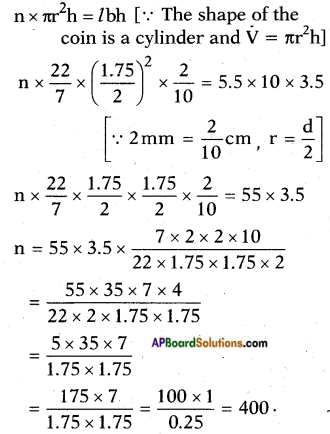∴ 400 silver coins are needed.

Question 7.
A vessel is in the form of an inverted cone. Its height is 8 cm. and the radius of its top is 5 cm. It is filled with water up to the rim. When lead shots, each of which is a sphere of radius 0.5 cm are dropped into the vessel, 1/4 of the water flows out. Find the number of lead shots dropped into the vessel.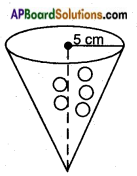Let the number of lead shots dropped = n
Then total volume of n lead shots = $$\frac{1}{4}$$ volume of the conical vessel.
Volume V = $$\frac{4}{3}$$πr3
= $$\frac{4}{3}$$ × $$\frac{22}{7}$$ × 0.5 × 0.5 × 0.5
Total volume of n – shots
= n × $$\frac{4}{3}$$ × $$\frac{22}{7}$$ × 0.125
Cone:
Height, h = 8 cm
Volume, V = $$\frac{1}{3}$$ πr2h
= $$\frac{1}{3}$$ × $$\frac{22}{7}$$ × 5 × 5 × 8
= $$\frac{1}{3}$$ × $$\frac{22}{7}$$ × 200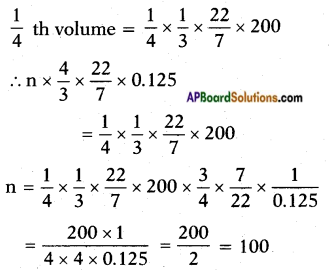∴ Number of lead shots = 100.Question 8.
A solid metallic sphere of diameter 28 cm is melted and recast into a number of smaller cones, each of diameter 4 $$\frac{d}{2}$$ cm and height 3 cm. Find the number of cones so formed.
Radius r = $$\frac{d}{2}$$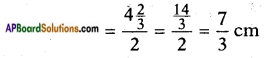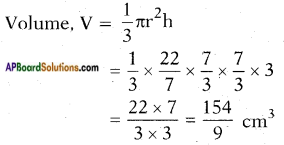Total volume of n-cones = n . $$\frac{154}{9}$$ cm3
Radius = $$\frac{d}{2}$$ = $$\frac{28}{2}$$ = 14 cm